# Random Distributions¶

Correctly called random variable, these random variable are usefull in hyperparameter tuning.

For each hyperparmeter, a range can be defined, that is, a statistical distribution, which makes the hyperparameter a random variable. This random variable will defined what value the hyperparameter is likely to take.

Let’s explore the hyperparameter distributions, by plotting the following graph : - Probability distribution function (pdf) or probability mass function (pmf) - Cumulative distribution function (cdf) - Histogram of sampling.

## Plotting Each Hyperparameter Distribution¶

Let’s import plotting functions, and neuraxle hyperparameter classes.

:

from neuraxle.hyperparams.distributions import *
from neuraxle.hyperparams.space import HyperparameterSpace
from neuraxle.plotting import plot_histogram, plot_pdf_cdf, plot_distribution_space
import matplotlib.pyplot as plt
import numpy as np
%matplotlib inline

DISCRETE_NUM_BINS = 40
CONTINUOUS_NUM_BINS = 1000
NUM_TRIALS = 100000
X_DOMAIN = np.array(range(-100, 600)) / 100


## Discrete Distributions¶

• Here are now the discrete standard distributions, which sample discrete value or categories.

• For example, Boolean distribution gives either true or false.

### RandInt¶

:

discrete_hyperparameter_space = HyperparameterSpace({
"randint": RandInt(1, 4)
})

plot_distribution_space(discrete_hyperparameter_space, num_bins=DISCRETE_NUM_BINS)


randint: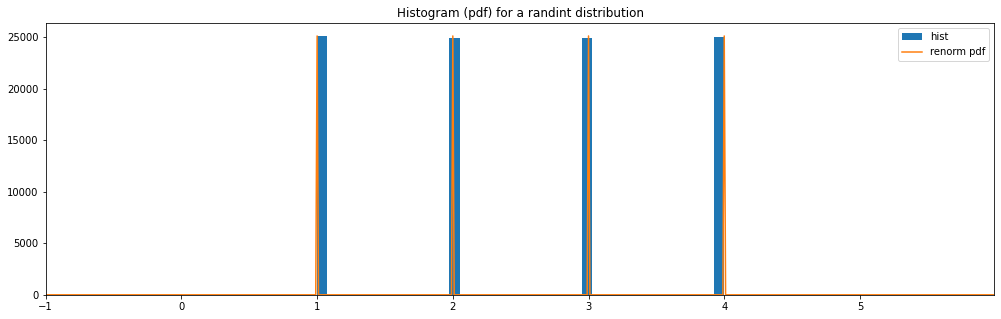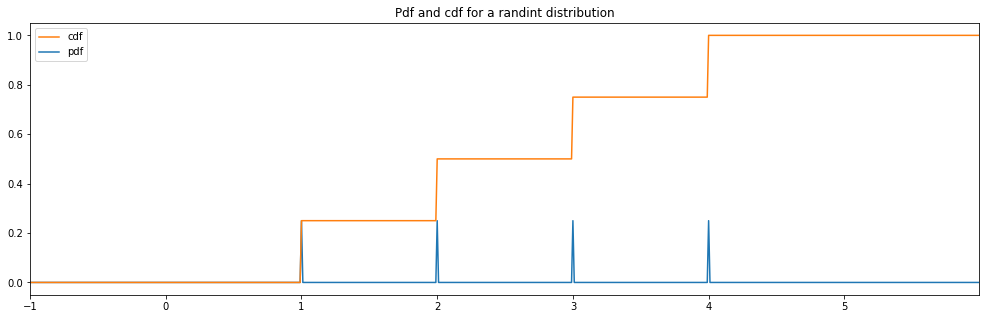### Boolean¶

:

discrete_hyperparameter_space = HyperparameterSpace({
"boolean": Boolean()
})
plot_distribution_space(discrete_hyperparameter_space, num_bins=DISCRETE_NUM_BINS)

boolean: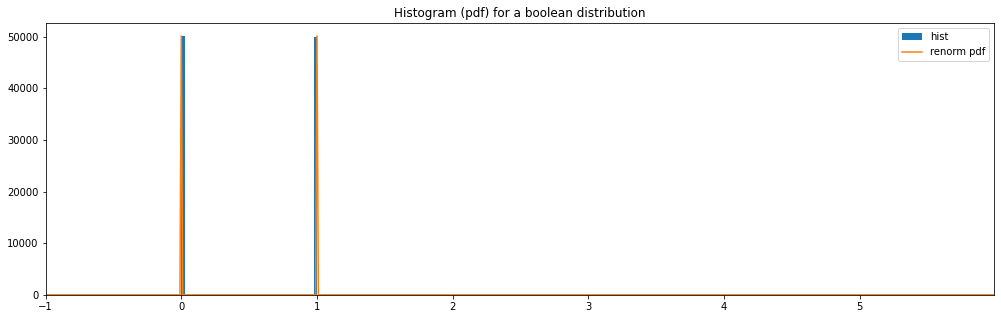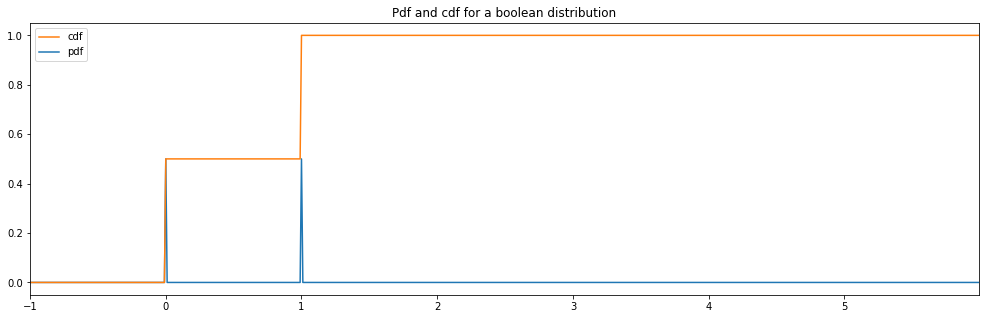### Choice¶

:

discrete_hyperparameter_space = HyperparameterSpace({
"choice": Choice([0, 1, 3])
})
plot_distribution_space(discrete_hyperparameter_space, num_bins=DISCRETE_NUM_BINS)

choice: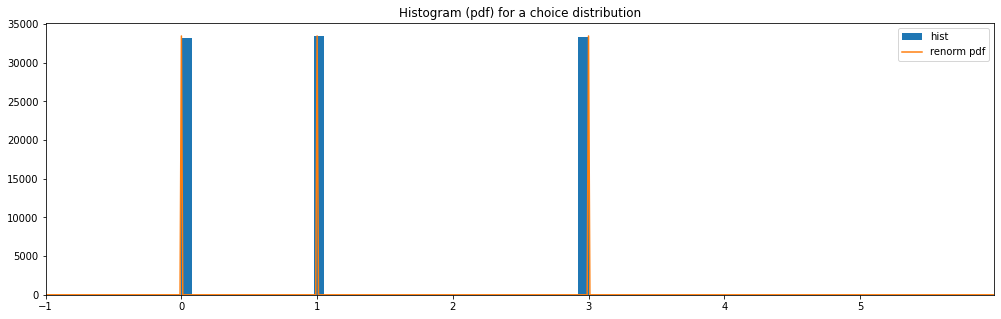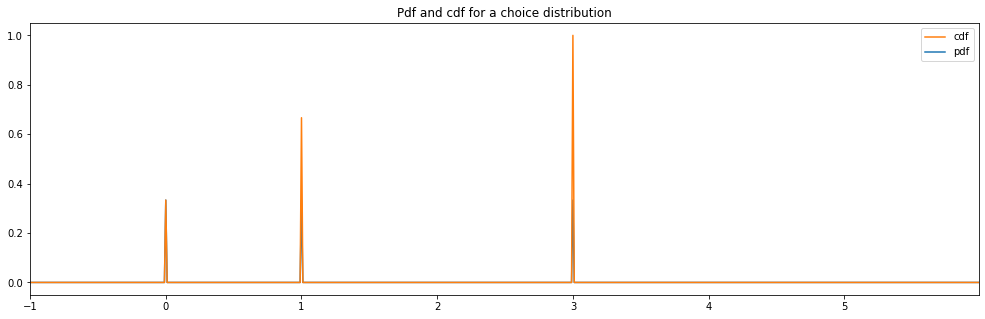### Priority Choice¶

:

discrete_hyperparameter_space = HyperparameterSpace({
"priority_choice": PriorityChoice([0, 1, 3])
})
plot_distribution_space(discrete_hyperparameter_space, num_bins=DISCRETE_NUM_BINS)

priority_choice: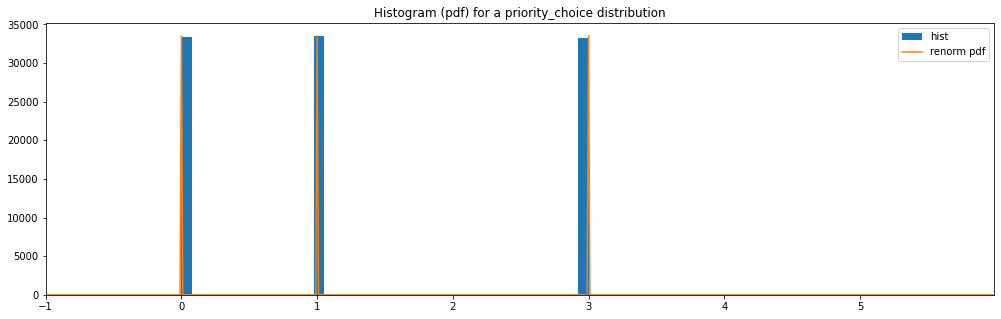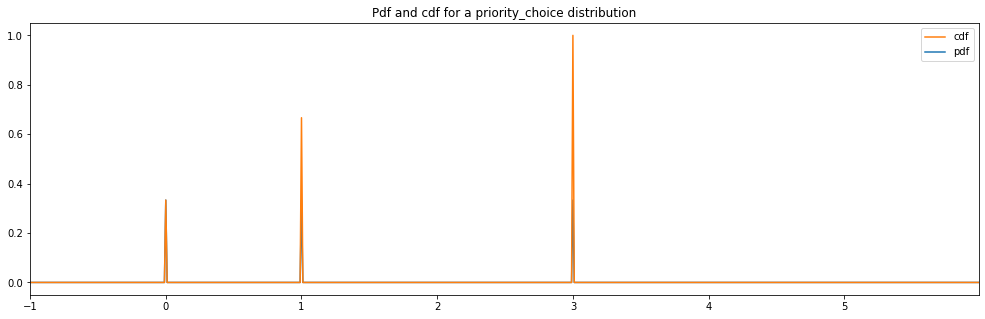## Continuous Distributions¶

• Here are now the continuous distributions, which sample a continuous range of values. Those are probably the ones you’ll most use.

### Continuous Uniform¶

:

continuous_hyperparameter_space = HyperparameterSpace({
"uniform": Uniform(2., 4.)
})
plot_distribution_space(continuous_hyperparameter_space, num_bins=CONTINUOUS_NUM_BINS)

uniform: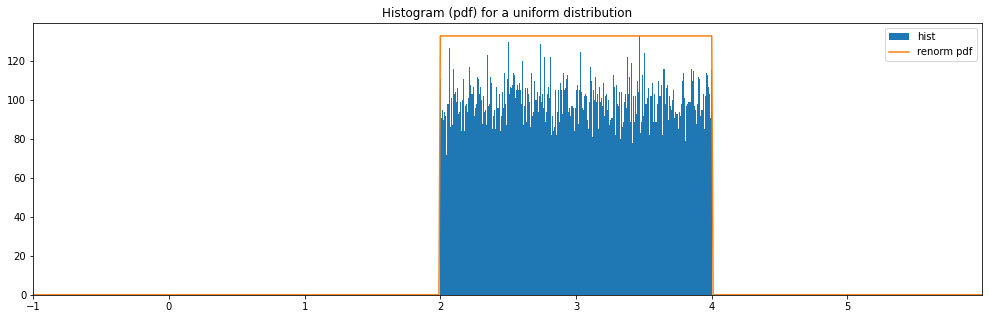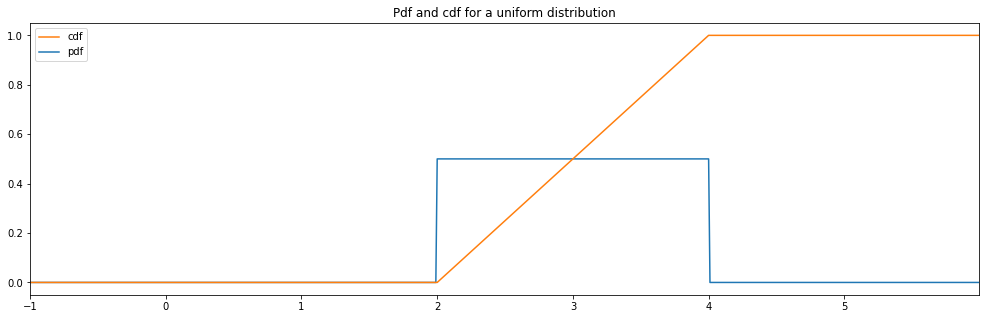### Continuous Loguniform¶

:

continuous_hyperparameter_space = HyperparameterSpace({
"loguniform": LogUniform(1., 4.)
})
plot_distribution_space(continuous_hyperparameter_space, num_bins=CONTINUOUS_NUM_BINS)

loguniform: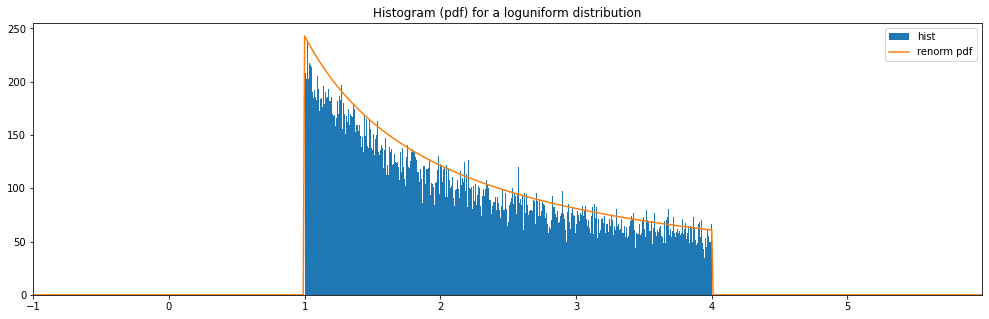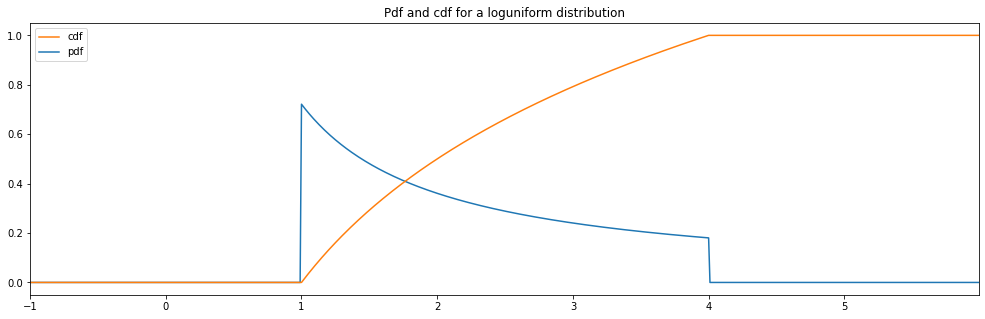### Continuous Normal¶

:

continuous_hyperparameter_space = HyperparameterSpace({
"normal": Normal(3.0, 1.0)
})
plot_distribution_space(continuous_hyperparameter_space, num_bins=CONTINUOUS_NUM_BINS)

normal: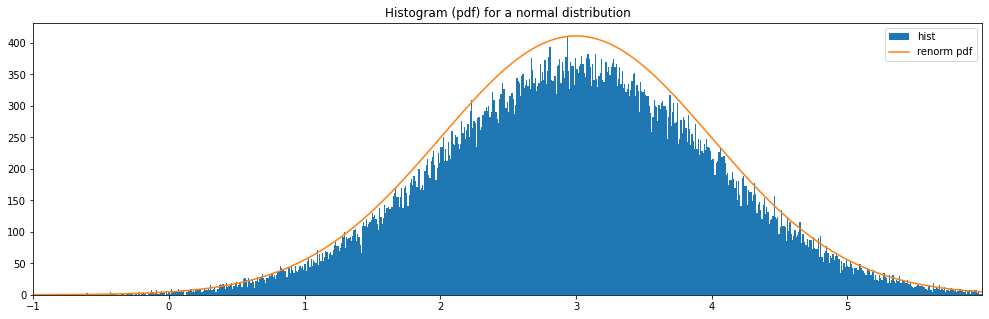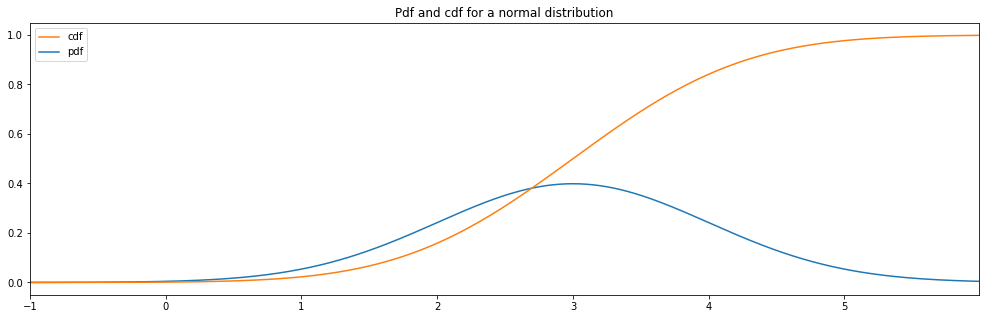### Continuous Lognormal¶

:

continuous_hyperparameter_space = HyperparameterSpace({
"lognormal": LogNormal(1.0, 0.5)
})
plot_distribution_space(continuous_hyperparameter_space, num_bins=CONTINUOUS_NUM_BINS)

lognormal: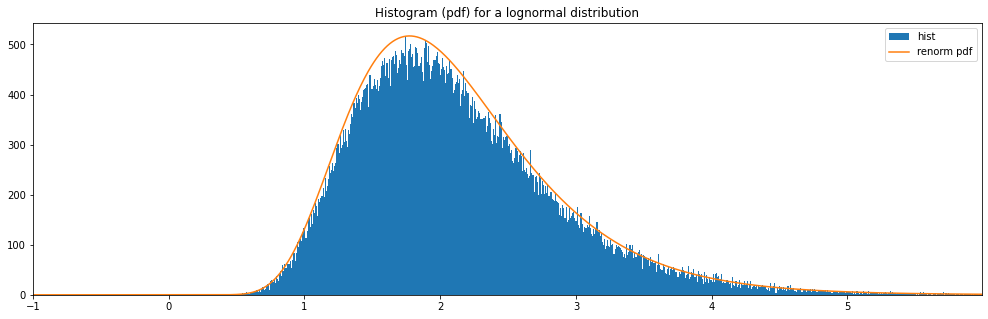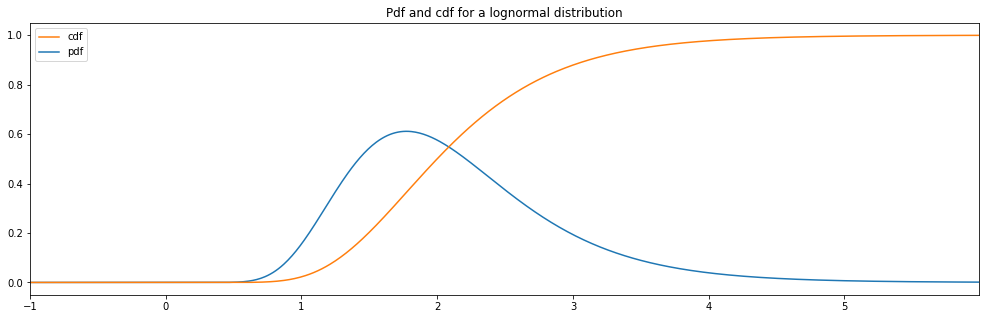### Continuous Normal Clipped¶

:

continuous_hyperparameter_space = HyperparameterSpace({
"normal_clipped": Normal(3.0, 1.0, hard_clip_min=1., hard_clip_max=5.)
})
plot_distribution_space(continuous_hyperparameter_space, num_bins=CONTINUOUS_NUM_BINS)

normal_clipped: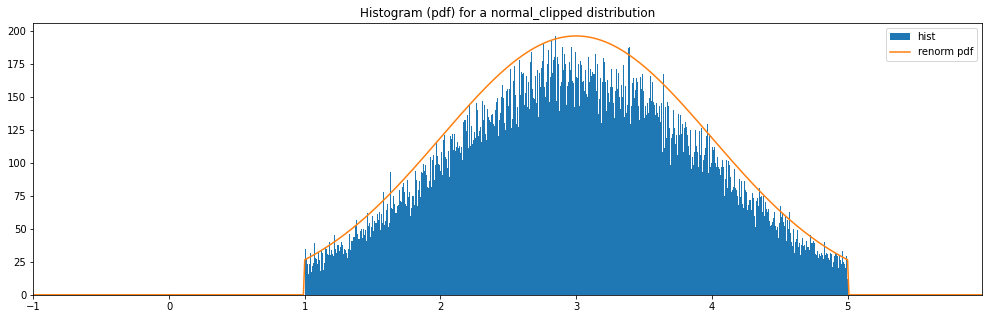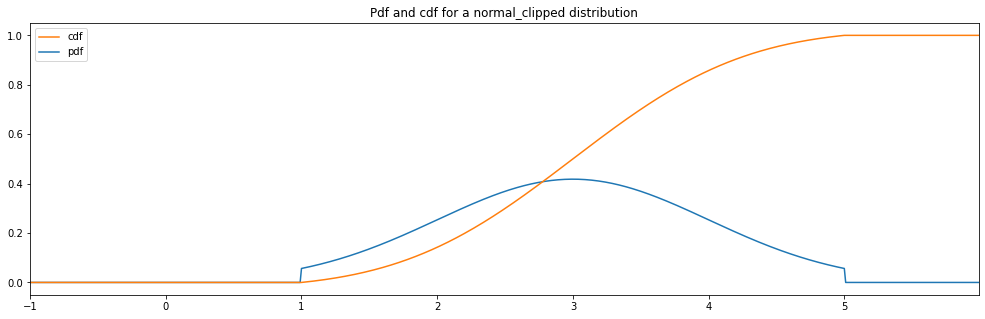### Continuous Lognormal Clipped¶

:

continuous_hyperparameter_space = HyperparameterSpace({
"lognormal_clipped": LogNormal(1.0, 0.5, hard_clip_min=2., hard_clip_max=4.)
})
plot_distribution_space(continuous_hyperparameter_space, num_bins=CONTINUOUS_NUM_BINS)

lognormal_clipped: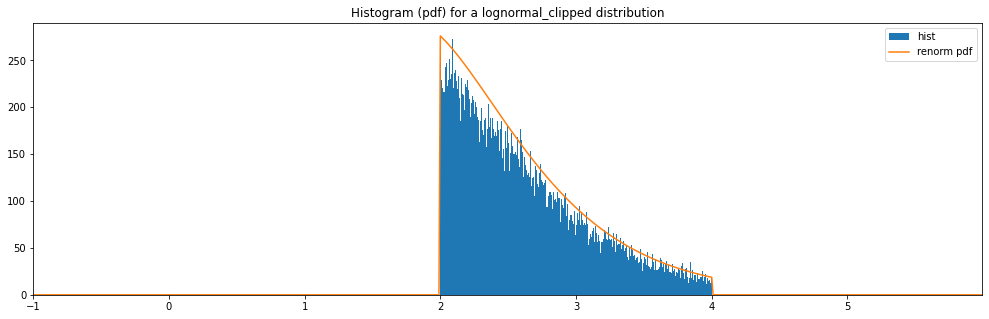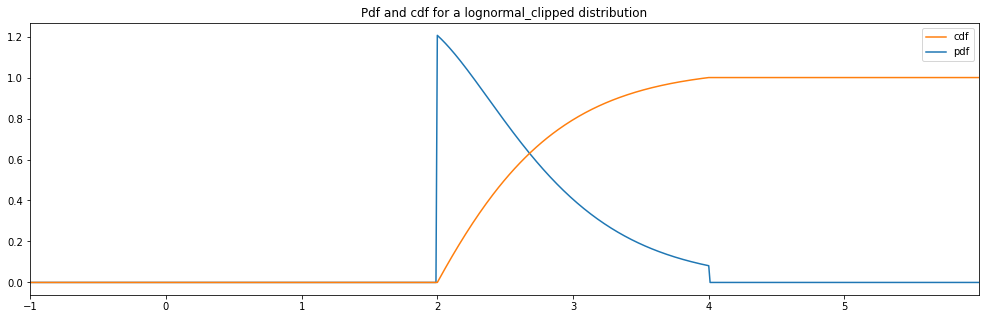## Quantized Hyperparameter Distributions¶

• Here are now the quantized hyperparameter distributions. Those are distributions that yield integers or other precise specific values.

• Also, notice how there are border effects to the left and right of the charts when we use Quantized(...) as a distribution wrapper to round the numbers.

• Those weird border effect wouldn’t appear if you’d limit the distribution to half numbers instead of plain number.

• Let’s say you have a Quantized(Uniform(-10, 10)): then the samples from approximately -9.5 to -8.5 are rounded to

• The bin of the number -9, but the values from -10 to -9.5 are rounder to the bin -10 and a half is missing, so the -10

• bin sees its values sampled half as often as -9. That explains the border effect, and you could fix it easily by taking the uniform range from -10.49999 to 10.49999.

### Quantized Uniform¶

:

quantized_hyperparameter_space = HyperparameterSpace({
"quantized uniform": Quantized(Uniform(1., 5.))
})
plot_distribution_space(quantized_hyperparameter_space, num_bins=DISCRETE_NUM_BINS)

quantized uniform: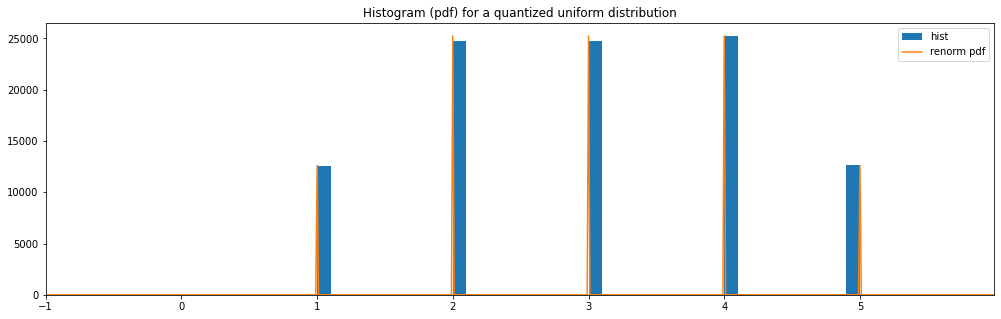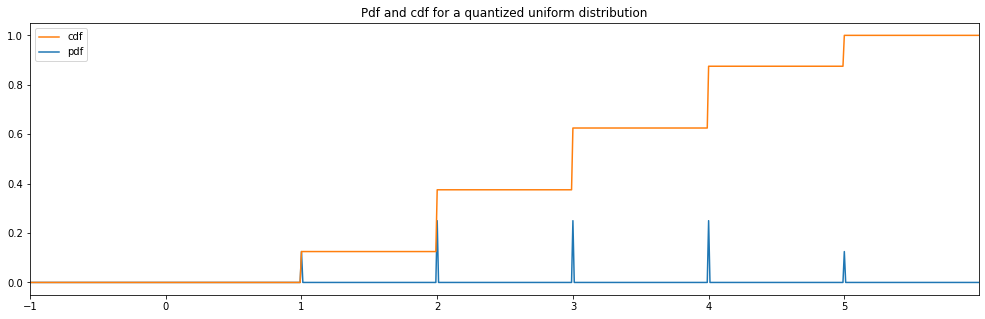### Repaired Quantized Uniform¶

:

quantized_hyperparameter_space = HyperparameterSpace({
"repaired quantized uniform": Quantized(Uniform(0.49999, 5.49999))
})

plot_distribution_space(quantized_hyperparameter_space, num_bins=DISCRETE_NUM_BINS)

repaired quantized uniform: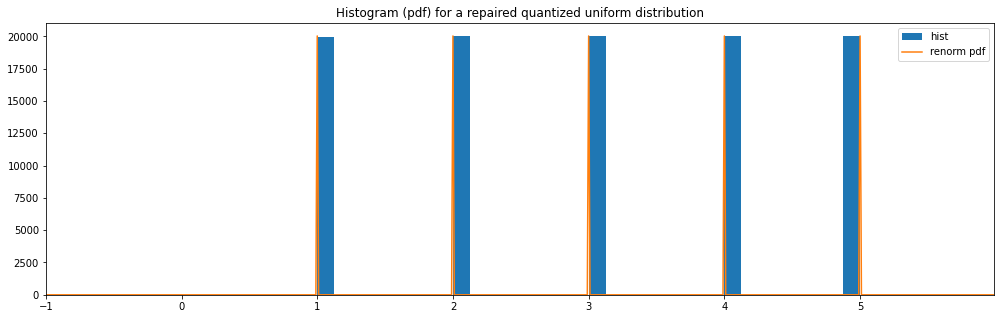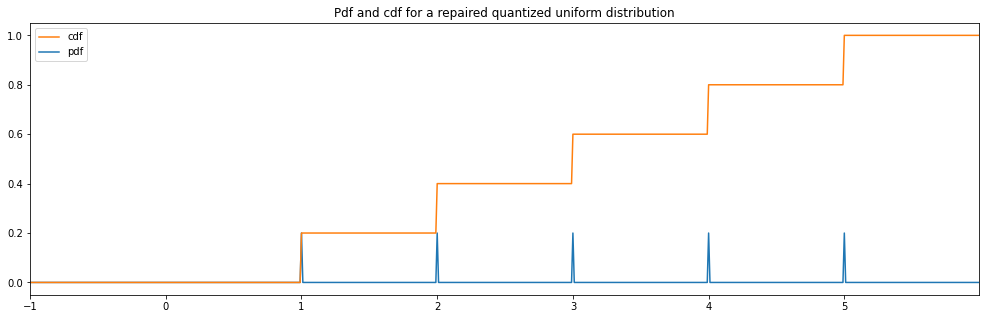### Quantized Log Uniform¶

:

quantized_hyperparameter_space = HyperparameterSpace({
"quantized loguniform": Quantized(LogUniform(1.0, 4.0))
})

plot_distribution_space(quantized_hyperparameter_space, num_bins=DISCRETE_NUM_BINS)

quantized loguniform: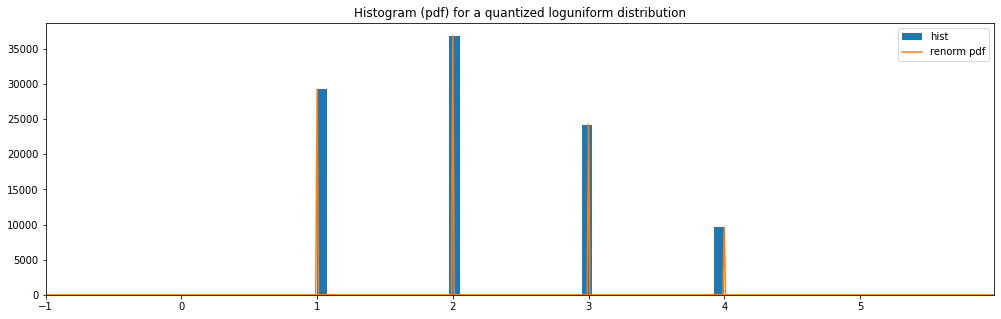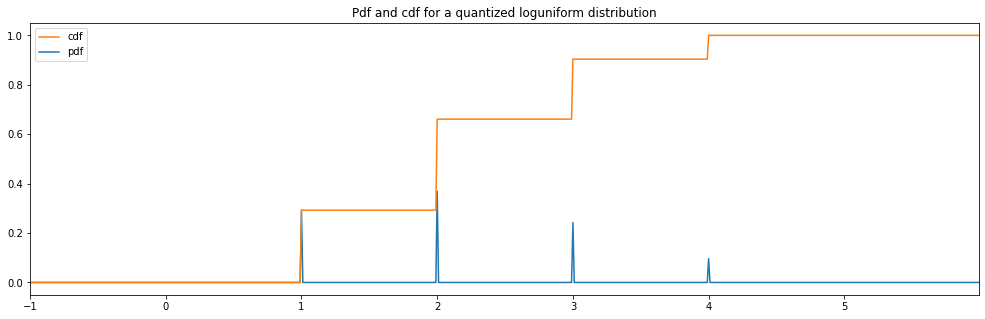### Quantized Normal¶

:

quantized_hyperparameter_space = HyperparameterSpace({
"quantized normal": Quantized(Normal(3.0, 1.0))
})

plot_distribution_space(quantized_hyperparameter_space, num_bins=DISCRETE_NUM_BINS)

quantized normal: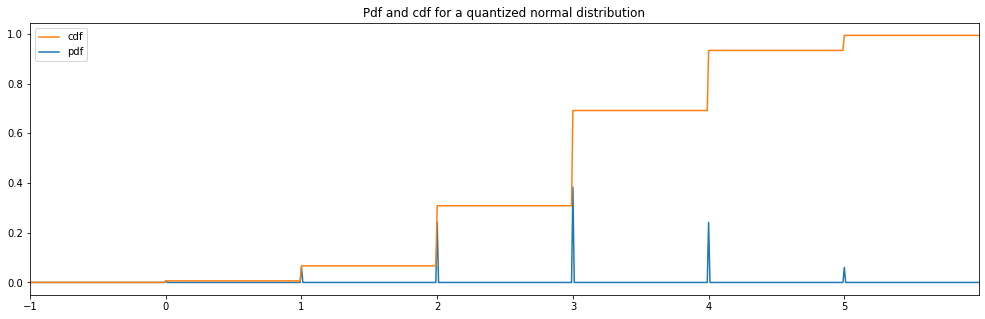### Quantized Lognormal¶

:

quantized_hyperparameter_space = HyperparameterSpace({
"quantized lognormal": Quantized(LogNormal(1.0, 0.5))
})
plot_distribution_space(quantized_hyperparameter_space, num_bins=DISCRETE_NUM_BINS)

quantized lognormal: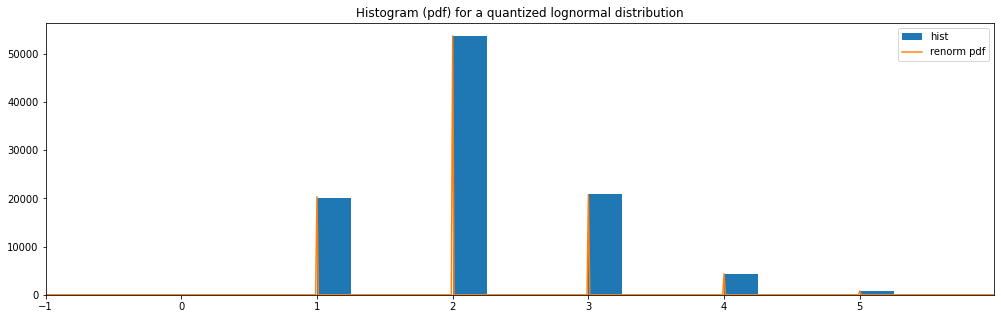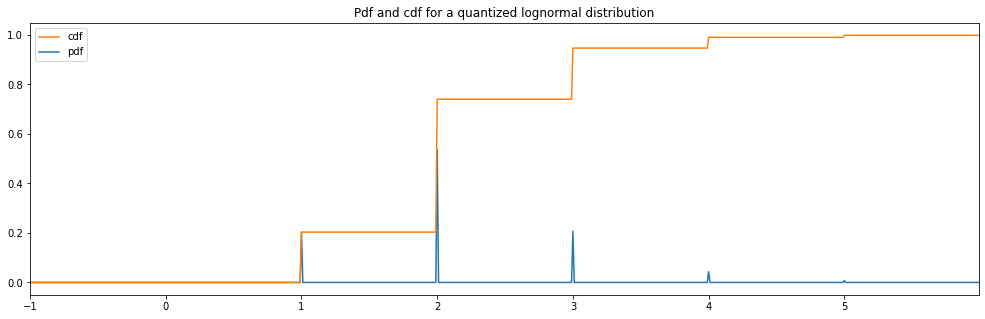Why would we limit our users to use just the pre-made distributions?! It’s easier than it looks! Here is for example how the RandInt distribution is coded:

:

class RandInt(DiscreteHyperparameterDistribution):
"""Get a random integer within a range"""

def __init__(self, min_included: int, max_included: int, null_default_value: int = None):
"""
Create a quantized random uniform distribution.
A random integer between the two values inclusively will be returned.

:param min_included: minimum integer, included.
:param max_included: maximum integer, included.
:param null_default_value: null default value for distribution. if None, take the min_included
:type null_default_value: int
"""
if null_default_value is None:
DiscreteHyperparameterDistribution.__init__(self, min_included)
else:
DiscreteHyperparameterDistribution.__init__(self, null_default_value)

self.min_included = min_included
self.max_included = max_included

def probabilities(self):
values = self.values()
return [1 / len(values) for _ in values]

def values(self):
return [i for i in range(self.min_included, self.max_included + 1)]

def rvs(self) -> int:
"""
Will return an integer in the specified range as specified at creation.

:return: an integer.
"""
return random.randint(self.min_included, self.max_included)

def pdf(self, x) -> float:
"""
Calculate the random int mass function value at position x.
:param x: value where the probability mass function is evaluated.
:return: value of the probability mass function.
"""

possible_values = set(range(self.min_included, self.max_included + 1))
if (isinstance(x, int) or x.is_integer()) and x in possible_values:
return 1 / (self.max_included - self.min_included + 1)

return 0.

def cdf(self, x) -> float:
"""
Calculate the random int cumulative distribution function value at position x.
:param x: value where the cumulative distribution function is evaluated.
:return: value of the cumulative distribution function.
"""
if x < self.min_included:
return 0.

if x > self.max_included:
return 1.

return (math.floor(x) - self.min_included + 1) / (self.max_included - self.min_included + 1)

def min(self):
"""
Calculate minimum value that can be sampled in the randint distribution.

:return: minimal value return from distribution.
"""
return self.min_included

def max(self):
"""
Calculate maximal value that can be sampled in the randint distribution.

:return: maximal value return from distribution.
"""
return self.max_included

def mean(self):
"""
Calculate mean value (also called esperance) of the random variable.

:return: mean value of the random variable.
"""
return (self.max_included + self.min_included) / 2

def var(self):
"""
Calculate variance value of the random variable.

:return: variance value of the random variable.
"""
return ((self.max_included - self.min_included + 1) ** 2 - 1) / 12


## Using Scipy Distributions¶

Scipy distributions are directly compatible with neuraxle ! Feel free to use them inside your hyperparameter spaces.

:

from neuraxle.base import Identity
from scipy.stats import norm, randint, gamma, uniform

hyperparams_space = HyperparameterSpace({
'rand_int_scipy': randint(low=2, high=5),
'gamma_scipy': gamma(0.2),
'uniform_scipy': uniform(0, 10)
})

print(hyperparams_space.rvs())

HyperparameterSamples([('rand_int_scipy', <scipy.stats._distn_infrastructure.rv_frozen object at 0x7fe8f3f3e290>), ('gamma_scipy', <scipy.stats._distn_infrastructure.rv_frozen object at 0x7fe8f2b28410>), ('uniform_scipy', <scipy.stats._distn_infrastructure.rv_frozen object at 0x7fe8f3f3e950>)])


## Creating your own distributions using scipy¶

You can also use scipy BaseCustomContinuousScipyDistribution, and BaseCustomDiscreteScipyDistribution to avoid a lot of boilerplate code like min, max, mean, and var methods.

### BaseCustomContinuousScipyDistribution¶

:

from neuraxle.hyperparams.scipy_distributions import BaseCustomContinuousScipyDistribution, BaseCustomDiscreteScipyDistribution

class Normal(BaseCustomContinuousScipyDistribution):
"""
Normal distribution that wraps a continuous scipy distribution <https://docs.scipy.org/doc/scipy/reference/generated/scipy.stats.rv_continuous.html#scipy.stats.rv_continuous>_
"""

def __init__(
self,
mean: float,
std: float,
hard_clip_min: float = None,
hard_clip_max: float = None,
null_default_value: float = None
):
super().__init__(
name='normal',
min_included=hard_clip_min,
max_included=hard_clip_max,
null_default_value=null_default_value
)

self.hard_clip_min = hard_clip_min
self.hard_clip_max = hard_clip_max
self.mean = mean
self.std = std

def _pdf(self, x) -> float:
"""
Calculate the Normal probability distribution value at position x.
:param x: value where the probability distribution function is evaluated.
:return: value of the probability distribution function.
"""

if self.hard_clip_min is not None and (x < self.hard_clip_min):
return 0.

if self.hard_clip_max is not None and (x > self.hard_clip_max):
return 0.

if self.hard_clip_min is not None or self.hard_clip_max is not None:
a = -np.inf
b = np.inf

if self.hard_clip_min is not None:
a = (self.hard_clip_min - self.mean) / self.std

if self.hard_clip_max is not None:
b = (self.hard_clip_max - self.mean) / self.std

return truncnorm.pdf(x, a=a, b=b, loc=self.mean, scale=self.std)

return norm.pdf(x, loc=self.mean, scale=self.std)

:

hyperparameter_space = HyperparameterSpace({
"normal": Normal(mean=0.0, std=2.0, hard_clip_min=-3.0, hard_clip_max=3.0)
})
print(hyperparameter_space.rvs())

HyperparameterSamples([('normal', -1.4669749077021386)])


### BaseCustomDiscreteScipyDistribution¶

:

from scipy.special import factorial

class Poisson(BaseCustomDiscreteScipyDistribution):
"""
Poisson distribution that inherits from scipy.stats.rv_discrete <https://docs.scipy.org/doc/scipy/reference/generated/scipy.stats.rv_histogram.html#scipy.stats.rv_histogram>_
"""

def __init__(self, min_included: float, max_included: float, null_default_value: float = None, mu=0.6):
super().__init__(
min_included=min_included,
max_included=max_included,
name='poisson',
null_default_value=null_default_value
)
self.mu = mu

def _pmf(self, x):
return math.exp(-self.mu) * self.mu ** x / factorial(x)


:

hyperparameter_space = HyperparameterSpace({
"poisson": Poisson(0.0, 10.0)
})
print(hyperparameter_space.rvs())

HyperparameterSamples([('poisson', 2)])